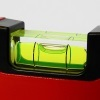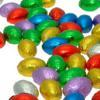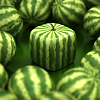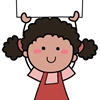Search by Topic

Filter by: Content type:
Age range:
Challenge level:

There are 12 NRICH Mathematical resources connected to NC-MultandDivFacts-R2-Y4, you may find related items under NC.

Broad Topics > NC > NC-MultandDivFacts-R2-Y4Satisfying Four Statements

Age 7 to 11 Challenge Level:

Can you find any two-digit numbers that satisfy all of these statements?Let Us Divide!

Age 7 to 11 Challenge Level:

Look at different ways of dividing things. What do they mean? How might you show them in a picture, with things, with numbers and symbols?Light the Lights Again

Age 7 to 11 Challenge Level:

Each light in this interactivity turns on according to a rule. What happens when you enter different numbers? Can you find the smallest number that lights up all four lights?Table Patterns Go Wild!

Age 7 to 11 Challenge Level:

Nearly all of us have made table patterns on hundred squares, that is 10 by 10 grids. This problem looks at the patterns on differently sized square grids.Times Tables Shifts

Age 7 to 11 Challenge Level:

In this activity, the computer chooses a times table and shifts it. Can you work out the table and the shift each time?The Remainders Game

Age 7 to 14 Challenge Level:

Play this game and see if you can figure out the computer's chosen number.Shape Times Shape

Age 7 to 11 Challenge Level:

These eleven shapes each stand for a different number. Can you use the number sentences to work out what they are?Multiplication Square Jigsaw

Age 7 to 11 Challenge Level:

Can you complete this jigsaw of the multiplication square?Multiples Grid

Age 7 to 11 Challenge Level:

What do the numbers shaded in blue on this hundred square have in common? What do you notice about the pink numbers? How about the shaded numbers in the other squares?Carrying Cards

Age 7 to 11 Challenge Level:

These sixteen children are standing in four lines of four, one behind the other. They are each holding a card with a number on it. Can you work out the missing numbers?Remainders

Age 7 to 14 Challenge Level:

I'm thinking of a number. My number is both a multiple of 5 and a multiple of 6. What could my number be?Zios and Zepts

Age 7 to 11 Challenge Level:

On the planet Vuv there are two sorts of creatures. The Zios have 3 legs and the Zepts have 7 legs. The great planetary explorer Nico counted 52 legs. How many Zios and how many Zepts were there?